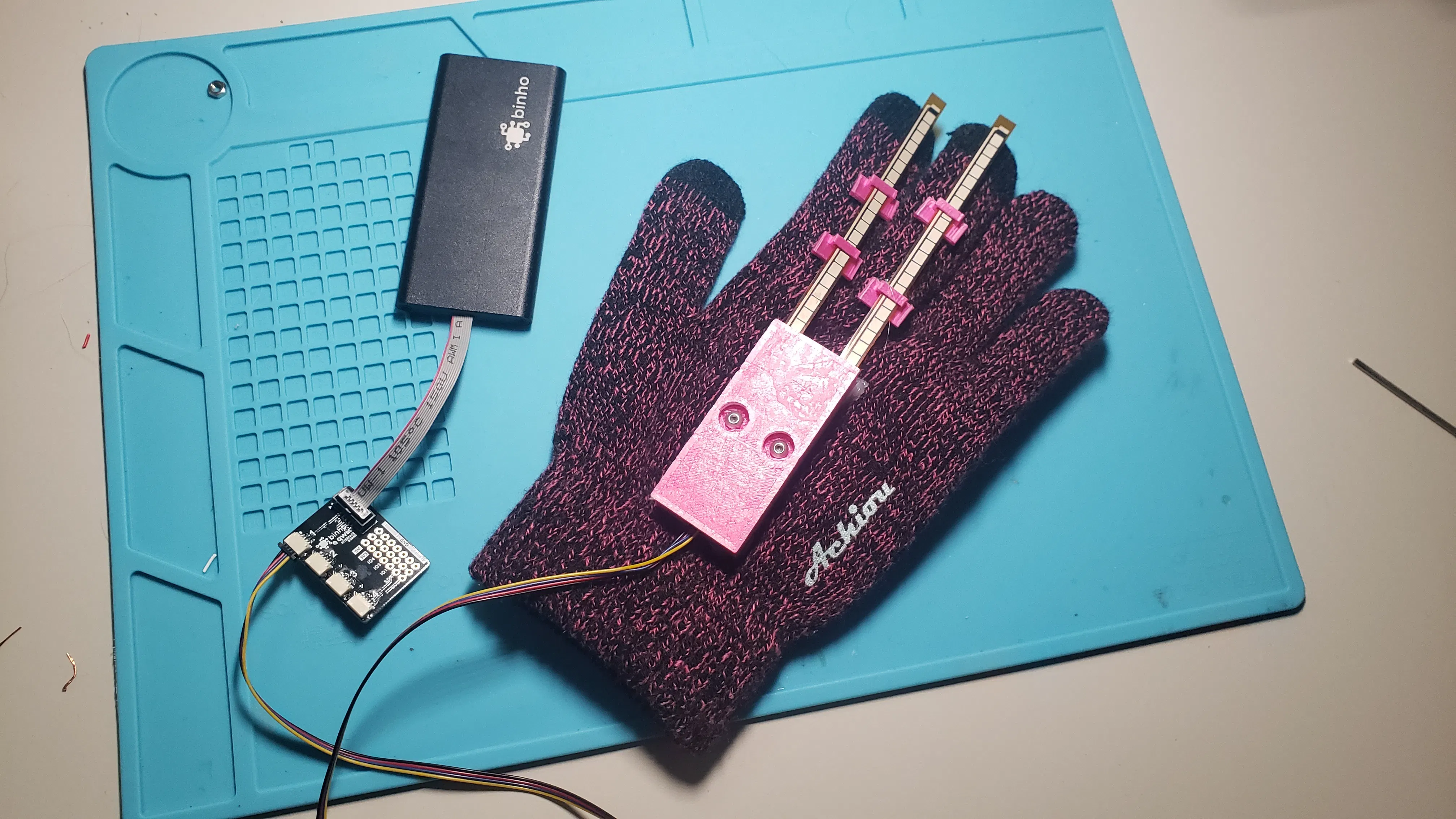Published © CC BY-NC-SA

# A Smart Glove Computer Mouse

This is a "Smart Glove" wearable computer mouse that can be used with any PC, Mac, or Linux computer.

IntermediateFull instructions provided1 hour3,487## Things used in this project

### Hand tools and fabrication machines3D Printer (generic)Hot glue gun (generic)

## Code

### Python Script

Python
The main Python script
```from binhoHostAdapter import binhoHostAdapter
from binhoHostAdapter import binhoUtilities
import time
import math
import pyautogui

pyautogui.FAILSAFE = True				# This will kill the script if the cursor touches a corner

def twos_comp(val, bits):
"""compute the 2's complement of int value val"""
if (val & (1 << (bits - 1))) != 0: # if sign bit is set e.g., 8bit: 128-255
val = val - (1 << bits)        # compute negative value
return val

#change this line to your com port number
binhoTesterCommPort = 'COM3'

# Tweak these to improve performance of Click detection
refreshInterval = 0.05
clickThreshold = 12000

enable_accel = True
enable_flex = True

# No Need to edit anything below here
finger1_isDepressed = False
finger2_isDepressed = False
finger1_clickFlag = False
finger2_clickFlag = False

finger1_maxValue = 0
finger1_minValue = 25000

finger2_maxValue = 0
finger2_minValue = 25000

print("SmartGlove V3 Script")

loop = True

# initialize the Binho host adapter for I2C communication
binhoTester.setNumericalBase('DEC')
binhoTester.setOperationMode(0, 'I2C')
binhoTester.setPullUpStateI2C(0, 'EN')
binhoTester.setClockI2C(0, 400000)

if enable_accel:

# put accelerometer in standby
binhoTester.writeByteI2C(0, 0x2A)
binhoTester.endI2C(0)
resp = result.split(" ")
ctrl_reg = int(resp) & ~(0x01)

binhoTester.writeByteI2C(0, 0x2A)
binhoTester.writeByteI2C(0, ctrl_reg)
binhoTester.endI2C(0)

# set scale
binhoTester.writeByteI2C(0, 0x0E)
binhoTester.endI2C(0)
resp = result.split(" ")

binhoTester.writeByteI2C(0, 0x0E)
binhoTester.writeByteI2C(0, 0x00)
binhoTester.endI2C(0)

#set datarate
binhoTester.writeByteI2C(0, 0x2A)
binhoTester.endI2C(0)
resp = result.split(" ")
ctrl_reg = int(resp) & 0xC7
ctrl_reg |= (0x01 << 3)

binhoTester.writeByteI2C(0, 0x2A)
binhoTester.writeByteI2C(0, 0x01)
binhoTester.endI2C(0)

while (loop == True):

if enable_accel:
# get values from accelerometer
binhoTester.writeByteI2C(0, 0x01)
binhoTester.endI2C(0)
resp = result.split(" ")
x_value = (int(resp) << 8 | int(resp))
y_value = (int(resp) << 8 | int(resp))
z_value = (int(resp) << 8 | int(resp))

x_signed = ((twos_comp(x_value, 16) >> 4))
y_signed = ((twos_comp(y_value, 16) >> 4))
z_signed = ((twos_comp(z_value, 16) >> 4))

x_gs = x_signed / 1024
y_gs = y_signed / 1024
z_gs = z_signed / 1024

mag = math.sqrt(x_gs**2 + y_gs**2 + z_gs**2)

#print('raw accel: [' + str(x_signed) + ', ' + str(y_signed) + ', ' + str(z_signed) +']')
#print('Gravity Vector: [' + str(x_gs) + 'g, ' + str(y_gs) + 'g, ' + str(z_gs) +'g]')
#print('Mag: ' + str(mag) + 'g')

# Move the cursor, scaled exponentially to tilt angle
if y_gs > 0.1:
x_speed = -400 * (y_gs**2)
elif y_gs < -0.1:
x_speed = 400 * (y_gs**2)
else:
x_speed = 0

if x_gs > 0.1:
y_speed = -400 * (x_gs**2)
elif x_gs < -0.1:
y_speed = 400 * (x_gs**2)
else:
y_speed = 0

pyautogui.moveRel(x_speed, y_speed, duration=0.1)

if enable_flex:
# read finger0 analog
binhoTester.startI2C(0, 0x90)
binhoTester.writeByteI2C(0, 0x01)
binhoTester.writeByteI2C(0, 0xC0)
binhoTester.writeByteI2C(0, 0x80)
binhoTester.endI2C(0)

binhoTester.startI2C(0, 0x90)
binhoTester.writeByteI2C(0, 0x00)
binhoTester.endI2C(0)

result = binhoTester.readBytesI2C(0, 0x90, 2)

resp = result.split(" ")

finger1 = int(int(resp) << 8 | int(resp))

if finger1 < finger1_minValue:
finger1_minValue = finger1
elif finger1 > finger1_maxValue:
finger1_maxValue = finger1

if finger1_isDepressed:
if finger1 > clickThreshold:
finger1_isDepressed = False
finger1_clickFlag = True
else:
if finger1 < clickThreshold:
finger1_isDepressed = True

if finger1_clickFlag:
finger1_clickFlag = False
#print('finger1: [currVal:' + str(finger1) + ', min:' + str(finger1_minValue) + ', max:' + str(finger1_maxValue)	+'] CLICK!')
pyautogui.click(button='right') # right click mouse
else:
#print('finger1: [currVal:' + str(finger1) + ', min:' + str(finger1_minValue) + ', max:' + str(finger1_maxValue)	+']')
pass

binhoTester.startI2C(0, 0x90)
binhoTester.writeByteI2C(0, 0x01)
binhoTester.writeByteI2C(0, 0xD0)
binhoTester.writeByteI2C(0, 0x80)
binhoTester.endI2C(0)

binhoTester.startI2C(0, 0x90)
binhoTester.writeByteI2C(0, 0x00)
binhoTester.endI2C(0)

binhoTester.startI2C(0, 0x90)
binhoTester.writeByteI2C(0, 0x00)
binhoTester.endI2C(0)

result = binhoTester.readBytesI2C(0, 0x90, 2)
resp = result.split(" ")

finger2 = int(int(resp) << 8 | int(resp))

if finger2 < finger2_minValue:
finger2_minValue = finger2
elif finger2 > finger2_maxValue:
finger2_maxValue = finger2

if finger2_isDepressed:
if finger2 > clickThreshold:
finger2_isDepressed = False
finger2_clickFlag = True
else:
if finger2 < clickThreshold:
finger2_isDepressed = True

if finger2_clickFlag:
finger2_clickFlag = False
#print('finger2: [currVal:' + str(finger2) + ', min:' + str(finger2_minValue) + ', max:' + str(finger2_maxValue)	+'] CLICK!')
pyautogui.click(button='left') # left click mouse
else:
#print('finger2: [currVal:' + str(finger2) + ', min:' + str(finger2_minValue) + ', max:' + str(finger2_maxValue)	+']')
pass

time.sleep(refreshInterval)

# close the connection to the Binho host adapter
binhoTester.close()
```

## Credits

### Cameron Coward

10 projects • 991 followers